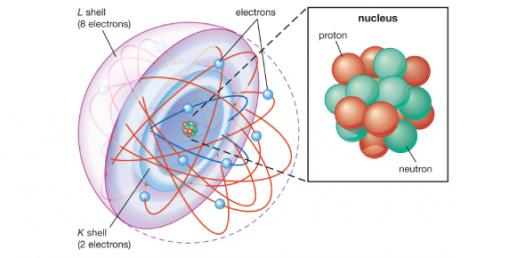# Chemistry Quiz: Trivia Questions On Atoms

Approved & Edited by ProProfs Editorial Team
At ProProfs Quizzes, our dedicated in-house team of experts takes pride in their work. With a sharp eye for detail, they meticulously review each quiz. This ensures that every quiz, taken by over 100 million users, meets our standards of accuracy, clarity, and engagement.
| Written by MeldaRoss
M
MeldaRoss
Community Contributor
Quizzes Created: 1 | Total Attempts: 344
Questions: 8 | Attempts: 345SettingsThis is about atoms. Pick the letter you think is correct.

• 1.

### Protons are

• A.

Are negatively charged

• B.

Orbit the nucleus

• C.

Are positively charged

• D.

1/2000 amu

C. Are positively charged
Explanation
Protons are positively charged particles found in the nucleus of an atom. They have a mass of approximately 1/2000 amu.

Rate this question:

• 2.

### Electrons are

• A.

Orbit the nucleus and negatively charged

• B.

Are positively charged and make up the nucleus

• C.

Take up most of the mass in the atom

• D.

Are the same as the atomic number

A. Orbit the nucleus and negatively charged
Explanation
Electrons are subatomic particles that revolve around the nucleus of an atom in specific energy levels or orbits. They carry a negative charge and are responsible for the majority of an atom's volume. The statement accurately describes the behavior and charge of electrons in an atom.

Rate this question:

• 3.

### The nucleus is

• A.

Is made up of protons and neutrons

• B.

Orbits the center proton

• C.

Is the coldest point in the atom

• D.

Surrounds the atom

A. Is made up of protons and neutrons
Explanation
The nucleus makes up most of the mass and is made up of protons and neutrons.

Rate this question:

• 4.

### The atomic number

• A.

Tells what can and what cannot move in an atom

• B.

Orbits the center proton

• C.

States how many spoolas are in the nucleus

• D.

Is the same as the amount of protons

D. Is the same as the amount of protons
Explanation
The atomic number of an atom refers to the number of protons in its nucleus. Protons are positively charged particles and are one of the fundamental components of an atom. The atomic number determines the element to which the atom belongs since each element has a unique number of protons. Therefore, the statement "the atomic number is the same as the amount of protons" is correct. It is important to note that the atomic number does not determine what can or cannot move in an atom, nor does it refer to the orbits of electrons or the number of spoolas in the nucleus.

Rate this question:

• 5.

### AMU stands for

• A.

Absolute Mass Unit

• B.

Atomic Making Unit

• C.

Atomic Mass Unit

• D.

Abraham's Mass Unit

C. Atomic Mass Unit
Explanation
AMU stands for Atomic Mass Unit. This unit is used to measure the mass of atoms and molecules. It is defined as 1/12th the mass of a carbon-12 atom. The atomic mass unit is commonly used in chemistry and physics to express the relative masses of atoms and molecules.

Rate this question:

• 6.

### If the atomic number is 9, the number of protons is

• A.

8

• B.

9

• C.

1

• D.

12

B. 9
Explanation
The atomic number represents the number of protons in an atom. Therefore, if the atomic number is 9, it means that there are 9 protons in the atom.

Rate this question:

• 7.

### If Uranium's atomic number is is 92 and it has 10 neutrons, how many protons does it have?

• A.

92

• B.

86

• C.

82

• D.

102

A. 92
Explanation
Uranium's atomic number represents the number of protons in its nucleus. Since the question states that Uranium's atomic number is 92, it means that Uranium has 92 protons. The number of neutrons is not relevant to determining the number of protons in an atom.

Rate this question:

• 8.

### If Aluminum has an atomic number of 13 and has 14 protons, what is its mass?

• A.

25

• B.

1

• C.

32

• D.

27Back to top